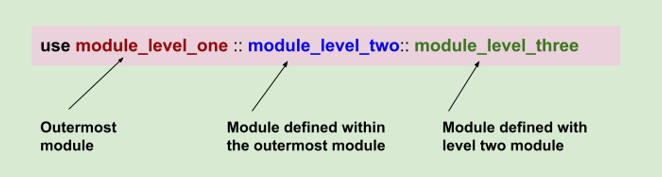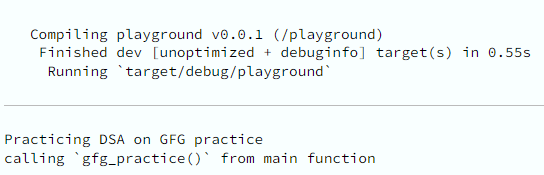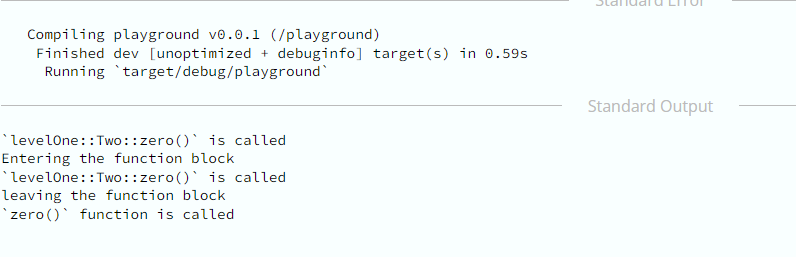Open in App
Not now

# Rust Use Declaration

• Last Updated : 27 Oct, 2022

In Rust, we have a concept of the ‘use‘ keyword. This keyword is used for importing or at times renaming items from other different modules or crates. Use the keyword to find its usage when we are required to import items or rename items from crates or modules in Rust programs. For precisely calling modules, the use of keywords is extremely helpful.

The benefit of using the use keyword is when all the items are present in the same module and it is required of them to be referred to in the code repeatedly due to certain programming logic. This allows us to not refer to the path to the code every time.Syntax:

use crate::deeply::nested::{
//        //Trait type is GfgTrait
};

fn main() {
…………… //calling geeks_for_geeks function
}

Here, we are invoking the use declaration function geeks_for_geeks. This declaration is used for binding the full path to the new names of our choice.

Example 1:

## Rust

 `use` `level_one::Two::zero ``as` `new_function; ` `fn` `zero() { ` `    ``println!(``"`zero()` function is called"``); ` `} ` ` `  `mod` `level_one { ` `    ``pub` `mod` `two { ` `        ``pub` `fn` `zero() { ` `            ``println!(``"`levelOne::Two::zero()` is called"``); ` `        ``} ` `    ``} ` `} ` ` `  `fn` `main() { ` `    ``new_function(); ` ` `  `    ``println!(``"Entering the function block"``); ` `    ``{ ` `        ``use` `crate``::level_one::two::zero; ` `        ``zero(); ` ` `  `        ``println!(``"leaving the function block"``); ` `    ``} ` ` `  `    ``zero(); ` `} `

Output:Explanation:

In this sample, we are using three modules named level_one, Two, and zero respectively. With the help of the use keyword, we are binding the three modules as ‘new_function‘. We have nested the two public functions in the module (mod level_one) and inside the zero function, we are printing the bound function. Inside the main function, we are calling the new_function which happens to be the nested function module i.e. equivalent to level_one::Two::zero. Here, the zero() function shadows the outer zero function() since ‘use’ bindings have a local scope, and the shadowing of zero() is only in this block.

Example 2:

## Rust

 `pub` `mod` `geeks_for_geeks { ` `    ``pub` `mod` `gfg_careers {  ` `        ``pub` `mod` `gfg_website {  ` `            ``pub` `fn` `gfg_practice() {   ` `              ``println!(``"Practicing DSA on GFG practice"``); ` `            ``} ` `        ``} ` `    ``} ` `} ` `use` `geeks_for_geeks::gfg_careers::gfg_website; ` `fn` `main() { ` `    ``gfg_website::gfg_practice(); ` `    ``println!(``"calling `gfg_practice()` from main function"``); ` `}`

Output:Explanation:

In this example, we have seen the different modules and how they can be used conveniently using the use keyword. We have declared the module geeks_for_geeks and assigned it to be public(pub). Now, there are three levels: gfg_careers which is in level one (public), gfg_website(public) at level two, and gfg_practice(public) at level three. In level three, we used the println! macro. Now, to print the macro we have used the use keyword. Here, level two and level three are nested under level one (geeks_for_geeks). Finally, we call the function with the module and the function at level three and see the output on the screen.

Many shortcuts are supported in the Use declaration. They are as follows:

• Binding  paths list with common prefixes like:
```use a::b::{c:d::e};
mod a{
pub mod b {
pub mod c {
fn d{
mod d{
pub mod e{
// methods and statements
}}}}}}```
• Binding paths list with common prefix along with a prefix common to the parent module by using the self keyword. use p::q::{self, one,two}
mod p{
pub mod q {
pub fn one(self) { // methods and statements }
pub fn two(self) { // methods and statements }
}}
• Using a new local name via Rebinding the paths list
```use a::b::c as z;
mod a {
pub mod b{
pub fn c{
// statement and methods
}}}```

My Personal Notes arrow_drop_up
Related Articles